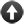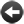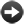§22.3. Phrases as values

Given any two kinds K and L, the kind "phrase K -> L" is now a kind. (This is meant to look like a mathematical function arrow.) For example, the phrase defined by

To decide which number is the square of (N - a number): ...

has the kind "phrase number -> number". Brackets and commas are used if the phrase combines several values, so

To decide which text is (T - text) repeated (N - a number) times: ...

has the kind "phrase (text, number) -> text". The word "nothing" is used if there are no values in, or no value out - thus

To decide which number is the magic target: ...

has kind "phrase nothing -> number", and

To dig (eastward - length) by (northward - length): ...

has the kind "phrase (length, length) -> nothing".

But how are we to get at these values? The answer is that we need to give a phrase a name in order to do so. For example:

To decide what number is double (N - a number) (this is doubling):
decide on N plus N.

This is the same syntax used to name rules, and the idea is the same. If we

try "showme doubling", the result is

phrase number -> number: doubling

The main thing we want to do with a phrase is to apply it. So:

showme doubling applied to 2;

produces

"doubling applied to 2" = number: 4

There are versions of "applied to" for phrases applied to 0 to 3 values:

(phrase nothing -> value) applied ... value

This phrase produces the result of applying the given phrase, which must be one which takes no values itself.

(phrase value -> value) applied to (value) ... value

This phrase produces the result of applying the given phrase, which must be one which takes one value itself.

(phrase (value, value) -> value) applied to (value) and (value) ... value

This phrase produces the result of applying the given phrase, which must be one which takes two values itself.

(phrase (value, value, value) -> value) applied to (value) and (value) and (value) ... value

This phrase produces the result of applying the given phrase, which must be one which takes three values itself.

So for example:

F applied
F applied to V
F applied to V and W
F applied to V and W and X

For phrases which do not produce a value, we use "apply":

apply (phrase nothing -> nothing)

This phrase causes the given phrase to be applied. It must be one which takes no values itself.

apply (phrase value -> nothing) to (value)

This phrase causes the given phrase to be applied. It must be one which takes one value itself.

apply (phrase (value, value) -> nothing) to (value) and (value)

This phrase causes the given phrase to be applied. It must be one which takes two values itself.

apply (phrase (value, value, value) -> nothing) to (value) and (value) and (value)

This phrase causes the given phrase to be applied. It must be one which takes three values itself.

Thus:

apply F;
apply F to V;
apply F to V and W;
apply F to V and W and X;Start of Chapter 22: Advanced PhrasesBack to §22.2. Descriptions as valuesOnward to §22.4. Default values for phrase kinds